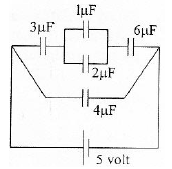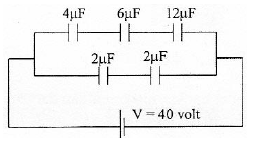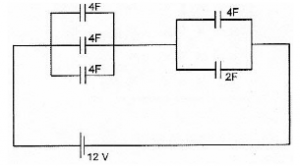# Electrical energy in capacitor circuits – problems and solutions

1. Determine the electrical energy in the circuit shown in the figure below (1 µF = 10-6 F)

Known :

Capacitor 1 (C1) = 3 µFCapacitor 2 (C2) = 1 µF

Capacitor 3 (C3) = 2 µF

Capacitor 4 (C4) = 6 µF

Capacitor 5 (C5) = 4 µF

Electric voltage (V) = 5 volts

Wanted : Electrical energy in circuits

Solution :

The equivalent capacitor :

Capacitor 2 and capacitor 3 are connected in parallel. The equivalent capacitor :

CA = C2 + C3 = 1 + 2 = 3 µF

Capacitor 1, capacitor A and capacitor 4 are connected in series. The equivalent capacitor :

1/CB = 1/C1 + 1/CA + 1/C4 = 1/3 + 1/3 + 1/6 = 2/6 + 2/6 + 1/6 = 5/6

CB = 6/5 µF

Capacitor B and capacitor 5 are connected in parallel. The equivalent capacitor :

C = CB + C5 = 6/5 + 4 = 6/5 + 16/4 = 24/20 + 80/20 = 104/20 = 5.2 µF

C = 5.2 x 10-6 Farad

Electrical energy in circuit :

E = ½ C V2 = ½ (5.2 x 10-6)(52) = (2.6 x 10-6)(25)

E = 65 x 10-6 Joule

2. Determine the electrical energy in capacitor circuits shown in figure below (1 µF = 10-6 F)

Known :

Capacitor 1 (C1) = 4 µFCapacitor 2 (C2) = 6 µF

Capacitor 3 (C3) = 12 µF

Capacitor 4 (C4) = 2 µF

Capacitor 5 (C5) = 2 µF

Electric voltage (V) = 40 volts

Wanted : Electrical energy in circuits

Solution :

The equivalent capacitor :

Capacitor 1, capacitor 2 and capacitor 3 are connected in series. The equivalent capacitor :

1/CA = 1/C1 + 1/C2 + 1/C3 = 1/4 + 1/6 + 1/12 = 3/12 + 2/12 + 1/12 = 6/12

CA = 12/6 = 2 µF

Capacitor 4 and capacitor 5 are connected in series. The equivalent capacitor :

1/CB = 1/C4 + 1/C5 = 1/2 + 1/2 = 2/2

CB = 2/2 = 1 µF

Capacitor A and capacitor B in parallel. The equivalent capacitor :

C = CA + CB = 2 + 1 = 3 µF

C = 3 x 10-6 Farad

Electrical energy in circuits :

E = ½ C V2 = ½ (3 x 10-6)(402) = (1.5 x 10-6)(1600)

E = 2400 x 10-6 = 2.4 x 10-3 Joule

3. Determine the energy stored in the electrical circuit shown in the figure below.

Known :

Capacitor 1 (C1) = 4 FCapacitor 2 (C2) = 4 F

Capacitor 3 (C3) = 4 F

Capacitor 4 (C4) = 4 F

Capacitor 5 (C5) = 2 F

Electric voltage (V) = 12 volts

Wanted : Electrical energy in circuits

Solution :

The equivalent capacitor :

Capacitor 1, capacitor 2 and capacitor 3 are connected in parallel. The equivalent capacitor :

CA = C1 + C2 + C3 = 4 + 4 + 4 = 12 F

Capacitor 4 and capacitor 5 are connected in parallel. The equivalent capacitor :

CB = C4 + C5 = 4 + 2 = 6 F

Capacitor A and capacitor B are connected in series. The equivalent capacitor :

1/C = 1/CA + 1/CB = 1/12 + 1/6 = 1/12 + 2/12 = 3/12

C = 12/3 = 4 Farad

Electrical energy in circuits :

E = ½ C V2 = ½ (4)(122) = (2)(144)

E = 288 Joule